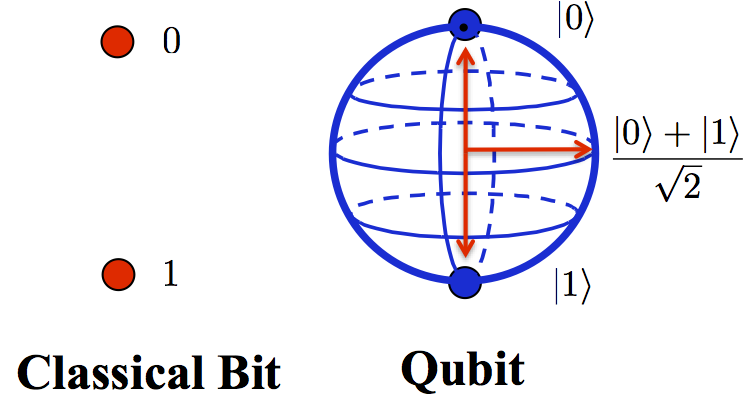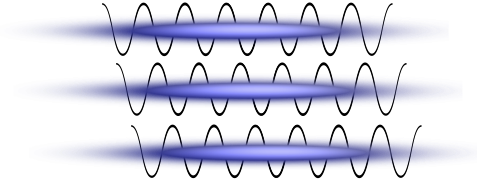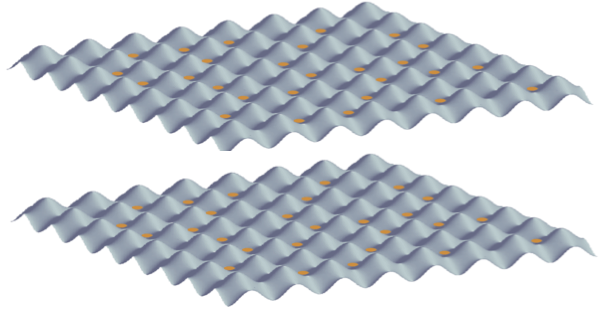## Measurement of Many-body Entanglement

What is entanglement?

Entanglement is a fundamental property of microscopic systems that are described by quantum mechanics. The first important ingredient for systems to be entangled is that they must first exist in a so-called quantum superposition of different states: for microscopic objects, it is possible for the system to take on more than one state at once (for example, where a classical computer stores information as "bits" which are either a zero or a one, a quantum computer can store information as "qubits", which can exist in a superposition of being both zero and one at once).In simple terms, if two objects are entangled it implies that they are each in a quantum superposition of different possible states, but that they are linked so that measuring one provides information about the other. For example, if we had two microscopic "coins" where each had two states - "heads" or "tails", then in quantum mechanics, they can be put in a superposition state so that each coin is simultaneously "heads" and "tails" at the same time. If we entangle the coins, then we can set up an odd situation in which whenever we measure whether the coins are "heads" or "tails", the outcomes are always linked. For example, we could set it up so that both are initially in a superposition of heads or tails, but if we measure the first coin coin (by looking to see if it is showing "heads" or "tails"), then whatever outcome we observe, we immediately know that the second coin will be in the same state.

This is phenomenon is strange, because even if we separate the coins, the measurement of the first coin affects the outcome of measuring the second coin. Einstein and other pioneers of studying such microscopic physics were very uncomfortable with the implications of this - he referred to this behaviour as "spooky action at a distance". In 1964, a physicist called John Bell showed that the correlations that could be produced for microscopic particles (as described by quantum mechanics) went beyond anything that was possible if made two seemingly reasonable assumptions: (i) that the measurement outcomes for the "coins" are predetermined before the measurement (so-called "realism" of the outcomes), and (ii) that measurement of one coin doesn't affect measurement of the other (this is called "locality", as the "coins" can be separated by long distances, so if measuring one affects the other then the effects would be non-local). When the correlations violate the bounds where these two assumptions hold together, we say that a system is "entangled", and it really implies that these systems behave in a way that is very different from our usual intuition. Beginning in the 1980s, such entanglement has been demonstrated for a variety of setups with few-particle systems of photons and atoms. It is not clear from quantum mechanics which of the two assumptions should be violated, but there are many theories that try to explain the results in terms of violation of one or the other. For a more detailed overview and further references, see, e.g., the Wikipedia article on Bell's theorem.

What about measuring many-body entanglement?Entanglement is also an important property for many-particle systems, e.g., as are found in quantum simulators. A lot of research has been done in the last ten years studying entanglement as it occurs in such systems, and understanding how it is linked to important many-body properties. Entanglement also underlies the complexity of simulating quantum systems on a classical computer, because most current generic simulation algorithms for many-body dynamics have to assume relatively weak entanglement in order to make the computations possible. It would thus be interesting and important to demonstrate rapid build-up of entanglement in experiments with quantum simulators, as part of characterising how these quantum simulators could begin to compete with classical computations.Together with collaborators in Austria, we have now proposed a scheme that would make use of state-of-the-art experimental techniques to measure entanglement in a many-body system of cold atoms in optical lattices. These techniques involve preparing multiple copies of the states, and then determining the entanglement by first coupling the copies in a careful way, then making measurements of local particle numbers in each site of each copy. They also provide a means to partially verify the accuracy of quantum simulators by directly comparing the quantum states prepared in each copy.

• Synopsis of our article and a related article by D. Abanin and E. Demler, in APS Physics
• A. J. Daley, H. Pichler, J. Schachenmayer, and P. Zoller, Measuring entanglement growth in quench dynamics of bosons in an optical lattice, Phys. Rev. Lett. 109, 020505 (2012). (arXiv:1205.1521)
• News article from the Institute for Quantum Optics and Quantum Information of the Austrian Academy of Sciences (in German).
• More on quantum simulators and quantum gases